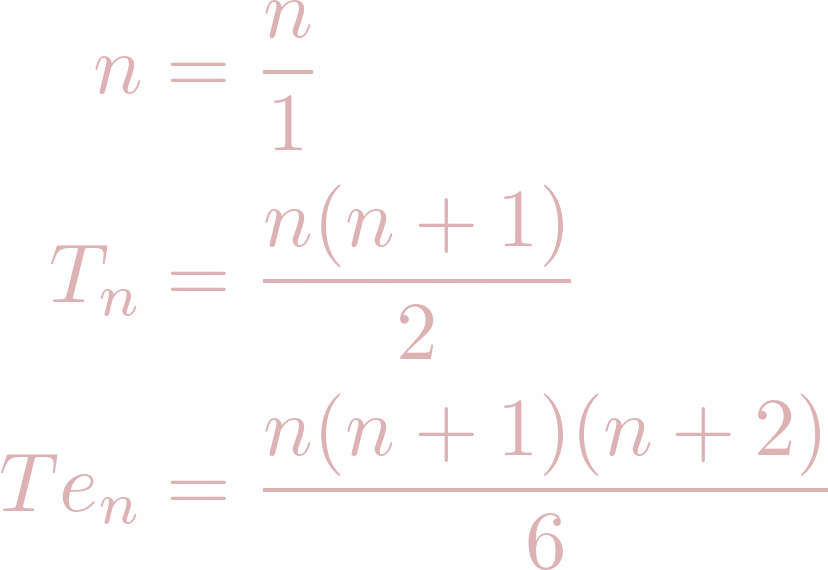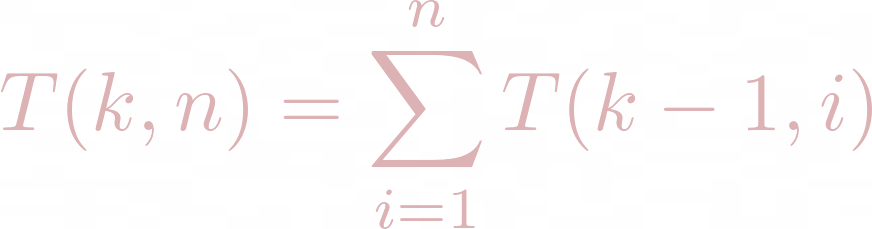Search

# Fun with the Dot Product (Math = Patterns)

Updated: Nov 22, 2021

Imagine this. You have a terribly strong desire to learn as much math as you can. You know that people do research in math, whatever that actually means. What you think it means is playing around with something mathematical until you discover something cool. So you try to do that. You're only in AP Calc, but sometimes you miss your history class and somehow end up in the Linear Algebra class that more advanced students get to take. You learn about vectors, which are big lists of numbers (4,2,7,1), and you learn about the dot product.

That awesome dot product! If you have two vectors of the same length, like v = (1,2,5) and u = (-1,2,2), then their dot product is found by multiplying each pair of numbers in the same spot, and adding it all up. So we get

1*(-1) = -1

2*2 = 4

5*2 = 10

v.u = -1 + 4 + 10 = 13

The general formula for the dot product of two vectors

v = (a1, a2, a3, ..., an), u = (b1, b2, b3, ..., bn)

is

v.u = a1b1 + a2b2 + a3b3 + ... + anbn

The dot product is very important in Linear Algebra, but remember that you're getting bits and pieces and you've never seen such advanced math before. So instead of exploring the geometry of the dot product, you begin to just look for patterns. You choose a vector, and you FLIP IT, then take that dot product. Why not?

(1).(1) = 1

(1,2).(2,1) = 2 + 2 = 4

(1,2,3).(3,2,1) = 3 + 4 + 3 = 10

(1,2,3,4).(4,3,2,1) = 4 + 6 + 6 + 4 = 20

Ok, so we get a sequence of numbers! 1,4,10, 20. What do these numbers mean? And if we take the general vector of length n, can we figure out what (1,2,3,...,n).(n, n-1, n-2, ..., 1) is? Well we can certainly write out what it is. Thankfully in Calc 1, we're talking about sequences and series, so we know how to use summation notation.The venerable Gauss taught usand another handy formula we've picked up is the sum of squares:We can use these to evaluate our sum for the dot product!Awesome! Let's skip the shtick, this doe-eyed person was me back at the beginning of my math career, right when I was discovered how unbelievably cool math research was. My sophomore year at JMU, I felt a bit more familiar with what math research even was, after doing an REU at Michigan State University on a wonderful topic in combinatorics. Finding a GENERAL FORMULA for anything was probably the height of success in my eyes back then.

So after figuring this general formula out, I was dying to find out more. I searched the sequence on OEIS.org (which is an amazing resource for any recreational mathematicians/combinatorialists) and discovered that this is a sequence called the Tetrahedral numbers.

This name comes from a geometric problem that GeeksForGeeks demonstrates quite well:Suppose you are stacking spheres to build a tetrahedron of height n. For n = 1, this is just 1 sphere. For n = 2, you have a base of three spheres and then one on top. For n = 3, we can just take our tetrahedron for n = 2, and put a bigger base with 6 spheres underneath. This actually demonstrates another way to define the tetrahedral numbers. Let's denote them by Te_n.We already have these two definitions:

The geometric point of view shows us a recursive definition. We can always take the tetrahedron for n and lift it up and put a base underneath it to get a tetrahedron for n+1. How many balls with will be in the base?

n = 1 ----- Base has 1 balls

n = 2 ----- Base has 3 balls

n = 3 ----- Base has 6 balls

n = 4 ----- Base has 10 balls

Another sequence! Notice that the base is a triangle. So there's no surprise this sequence of numbers is dubbed the Triangular numbers. Either from OEIS or from GeeksForGeeks again, we get a nice visual and formula. Denoting the triangular numbers by T_n:So we can work out our recursive definition of the tetrahedral numbers as:Sidetracking from the dot product stuff for a bit, there is another thing this recursive definition shows us. If we keep expanding it out, we see the Tetrahedral number Te_n is the sum of the first n triangular numbers. Similarly, the triangular number T_n is the sum of the first n natural numbers. We can even go further and say that the natural number n is the sum of 1, n times.

In symbols,Let's replace the sums with the general formulas:And we see a general pattern appear! Let's define T(k,n) to be the n^th k-dimensional triangular number. That is T(1,n) = n and T(2,n) = T_n and T(3,n) = Te_n. Specifically,Then we can conjecture from the general formulas for n, T_n, and Te_n thatSee if you can prove this! Try induction on k...